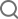Search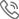+86-0755-28772795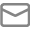sales@halnziye.com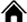> Home>Contact Us>Latest News> Thermal Resistance & Thermal Conductance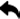Return to List

Thermal Resistance & Thermal Conductance

Introduction

Thermal resistance (R) and thermal conductance (C) of the materials are reciprocals of one another and can be

derived from thermal conductivity (k) and the thickness of the materials.

(1)

k=q L∕ΔT

Where,

L – Thickness of the specimen (m)

T – Temperature (K)

q – Heat flow rate (W/m2)

R-value – Thermal Resistance

Thermal resistance is the temperature difference, at steady state, between two defined surfaces of a material or

construction that induces a unit heat flow rate through a unit area, K⋅m2/W. According to this definition and Equation 1,

Equation 2, therefore, can be obtained.As indicated in Equation 2, the value of the thermal resistance can be determined

by dividing the thickness with thermal conductivity of the specimen.

(2)

R=ΔT/q=L/K

C-value – Thermal Conductance

Thermal conductance is the time rate of steady state heat flow through a unit area of a material or construction induced

by a unit temperature difference between the body surfaces, in W/m2⋅K. C-value, hence, is the reciprocal of the R-value and can be expressed as Equation (3).

(3)

C=q/ΔT=l/R=k/L

Consequently, the value of the thermal conductance can be calculated by dividing the thermal conductivity with the thickness

of the specimen.×
• Products

• Solutions

• Support